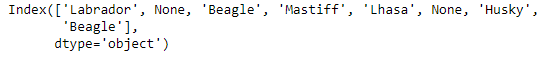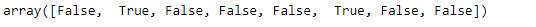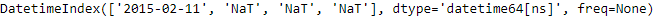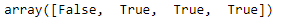GeeksforGeeks App
Open AppBrowser
Continue

# Python | Pandas Index.isnull()

Python is a great language for doing data analysis, primarily because of the fantastic ecosystem of data-centric python packages. Pandas is one of those packages and makes importing and analyzing data much easier.

Pandas` Index.isnull()` function detect missing values. It return a boolean same-sized object indicating if the values are NA. NA values, such as None, numpy.NaN or pd.NaT, get mapped to True values. Everything else get mapped to False values. Characters such as empty strings ‘’ or numpy.inf are not considered NA values (unless you set pandas.options.mode.use_inf_as_na = True).

Syntax: Index.isnull()

Parameters : Doesn’t take any parameter.

Returns : A boolean array of whether my values are NA

Example #1: Use `Index.isnull()` function to check if any of the value in the Index is a `NaN` value.

 `# importing pandas as pd``import` `pandas as pd`` ` `# Creating the Index``idx ``=` `pd.Index([``'Labrador'``, ``None``, ``'Beagle'``, ``'Mastiff'``,``                   ``'Lhasa'``, ``None``, ``'Husky'``, ``'Beagle'``])``# Print the Index``idx`

Output :Now we check for the missing values in the Index.

 `# checks for missing values.``idx.isnull()`

Output :The function returned an array object having the same size as that of the index. `True` value means the index label was missing and `False` value means the index label was present.

Example #2: Use `Index.isnull()` function to check if the missing Datetime Indexes are considered `NaN` values or not.

 `# importing pandas as pd``import` `pandas as pd`` ` `# Creating the Datetime Index``idx ``=` `pd.DatetimeIndex([pd.Timestamp(``'2015-02-11'``),``                   ``None``, pd.Timestamp(''), pd.NaT])`` ` `# Print the Datetime Index``idx`

Output :Now we will check if the labels in the Datetime Index are present or missing.

 `# test whether the passed Datetime Index``# labels are missing or not.``idx.isnull()`

Output :As we can see in the output, the function has returned an array object having the same size as that of the Datetime Index. `True` value means the index label are missing and `False` value means the index label are not missing.

My Personal Notes arrow_drop_up